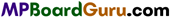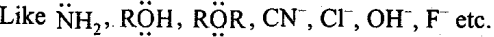These MP Board Class 11th Chemistry Notes for Chapter 7 Equilibrium help students to get a brief overview of all the concepts.

## MP Board Class 11th Chemistry Notes Chapter 7 Equilibrium

→ Equilibrium : Dynamic state in which velocities of forward reaction and backward reaction are equal.

→ Physical equilibrium : Equilibrium related to physical reactions.

→ Reversible reaction : The reaction which proceeds in forward and backward directions.

→ Chemical equilibrium : The state of a revesible reaction at which velocities of forward and backward reactions are same.→ Law of mass action: The rate of reaction of any substance is proportional to its active mass at constant temperature. The rate of chemical reaction is proportional to the product of active masses of reactants.

→ Equilibrium constant Kc = $$\frac{[\text { Product }]}{\text { [Reactant }]}$$

→ Relation between Kc and Kp : Kp = Kc (RT)Δn

→ Le-Chatelier’s principle : At equilibrium, when temperature, pressure or concentration of any system- is changed, the equilibrium* * shifts in the direction, so that effect of change is minimized.

→ Degree of ionisation: Ratio of number of molecules dissociated and total number of molecules.

→ Weak electrolyte : Electrolyte whose ionisation is less.

→ Ostwald’s dilution law: Degree of dissociations of weak electrolyte is proportional to square root of dilution.
Kα =  Kα = Cα2 or α = $$\sqrt{\frac{\mathrm{K}_{a}}{\mathrm{C}}}$$or α = $$\sqrt{\mathrm{K}_{\mathrm{a}} \mathrm{V}}$$
(1 – α) = 1 (for weak electrolyte) ,→ Arrhenius concept of acid-base: The substances producing H+ ions in aqueous solution are acids and the substances which produce OH- ions in aqueous solution are bases.

→ Bronsted-Lowry concept of acid-base : Proton donors are acids while proton acceptors are bases.

→ Lewis theory: Bases are the species which can donate lone pair of electrons while electron
pair acceptors are acids.

→ Ionic product of water : Kw = [H+][OH] = [H3O+][OH ] = 1 x 10-14

→ pH value : Negative logarithm value of molar concentration of H+ ion or H3O+ (in aqueous solution) as base 10 is called pH value.
pH = -log10[H+]

→ Solubility product: At constant temperature; product of molar concentration of ions in a saturated, solution of solute.

→ Henry’s law: The mass of gas dissolved in definite quantity of solvent at constant temperature is proportional to pressure of gas.
m x P or m = KP m = Mass of gas per unit volume
P = Gas pressure.

→ Salt formed by strong base and weak acid : CH3COONa, Na2CO3, Na3PO4, K2CO3 pH = 7 + $$\frac { 1 }{ 2 }$$ [pKa + log C]→ Salt formed by strong acid and weak base : CaCl2, NH4NO3, NH4Cl, (NH4)2SO4, AlCl3 PH = $$\frac { 1 }{ 2 }$$ [pKw – pKb – log C]

→ Salt formed by weak acid and weak base : CH3COONH4, (NH4)2CO3, Ca3(PO4)2

→ Lewis base : Species which possess lone electron pair.→ Lewis acid : Species whose central atom does not possess octet.
Like AlCl3, BF3, FeCl3, Ag+, H+, CO2, SO2, NH4, BCl3 etc.

→ Water is an amphoteric solution.